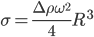# thesIt

##Arif

• #### 12:06:03 pm on February 19, 2010 | # | 0 Tags: angular velocity, density, equation, interfacial tension, spinning drop, wikipedia

Interfacial tension and centrifugal forces balanced at mechanical equilibrium.centrifugal-IFT equilibrium

Interfacial tension of any liquid that gives a shape very close to a cylinder at the equilibrium point, can be estimated using Vonnegut’s expression:$\sigma=\frac{\Delta\rho\omega^2}{4}R^3$

ω : angular velocity; (radians per second, degrees per second, revolutions per second)

Δρ : difference between two fluid: the less-dense and the dense